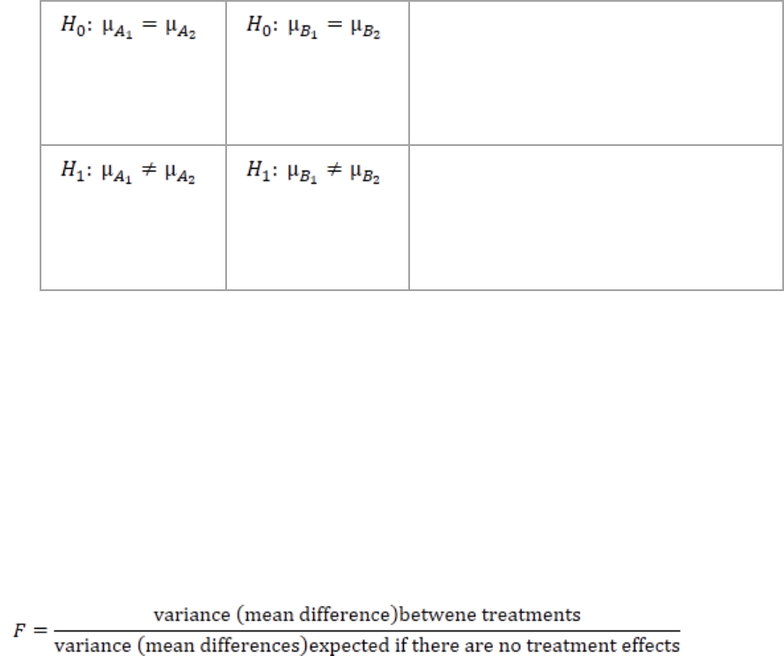# PSYC 2530 Chapter Notes - Chapter 14: Repeated Measures Design, Analysis Of Variance

38 views2 pages
School
Department
Course
Professor
Page:
of 2Ch. 14 - Two-Factor ANOVA
Tuesday, November 13, 2012
9:21 PM
PSYC 2530 Introductory Statistics
Chapter 14: Two-Factor Analysis of Variance (Independent Measures)
The mean differences among the levels of one factor are referred to as the main
effect of that factor. When the design of the research study is represented as a
matrix with one factor determining the rows and the second factor determining
the columns, then the mean differences among the rows describe the mean effect
of one factor, and the mean differences among the columns describe the main
effect for the second factor.
Hypotheses for Two-Factor ANOVA:
o
There is no interaction between factors A
and B. All of the mean differences
between treatment conditions are
explained by the main effects of the two
factors.
There is an interaction between factors.
The mean differences between treatment
conditions are not what would be
predicted from the overall main effects of
the two factors.
Interactions
o An interaction between two factors occurs whenever the mean differences
between individual treatment conditions, or cells, are different from what
would be predicted from the overall main effects of the factors.
o When the effect of one factor depends on the different levels of a second
factor, then there is an interaction between the factors.
o When the results of a two-factor study are presented in a graph, the existence
of nonparallel lines (lines that cross or converge) indicated an interaction
between the two factors.